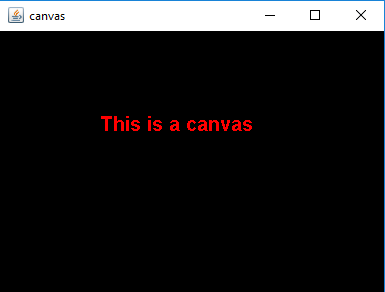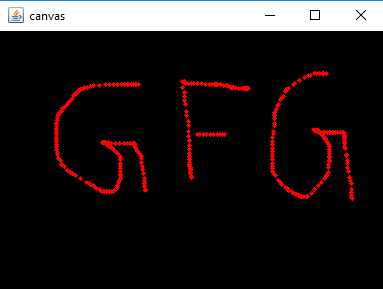# Java AWT | Canvas Class

Canvas class is a part of Java AWT. Canvas is a blank rectangular area where the user can draw or trap input from the user. Canvas class inherits the Component class.

Constructor of the Canvas class are :

1. Canvas(): Creates a new blank canvas.
2. Canvas(GraphicsConfiguration c): Creates a new canvas with a specified graphics configuration.

#### Commonly used Methods in Canvas Class

Method Explanation
addNotify() Creates the peer of the canvas.
createBufferStrategy(int n) Creates a new strategy for multi-buffering on this component.
createBufferStrategy(int n, BufferCapabilities c) Creates a new strategy for multi-buffering on this component with the required buffer capabilities
getBufferStrategy() Returns the BufferStrategy used by this component.
paint(Graphics g) paints this component.

Below programs illustrate the use of Canvas Class :

• Program 1: To create a canvas and paint the canvas.

 `// Java Program to create a to create ` `// a canvas and paint the canvas ` `import` `java.awt.*; ` `import` `javax.swing.*; ` `class` `canvas ``extends` `JFrame { ` ` `  `    ``// constuctor ` `    ``canvas() ` `    ``{ ` `        ``super``(``"canvas"``); ` ` `  `        ``// create a empty canvas ` `        ``Canvas c = ``new` `Canvas() { ` ` `  `            ``// paint the canvas ` `            ``public` `void` `paint(Graphics g) ` `            ``{ ` `                ``// set color to red ` `                ``g.setColor(Color.red); ` ` `  `                ``// set Font ` `                ``g.setFont(``new` `Font(``"Bold"``, ``1``, ``20``)); ` ` `  `                ``// draw a string ` `                ``g.drawString(``"This is a canvas"``, ``100``, ``100``); ` `            ``} ` `        ``}; ` ` `  `        ``// set background ` `        ``c.setBackground(Color.black); ` ` `  `        ``add(c); ` `        ``setSize(``400``, ``300``); ` `        ``show(); ` `    ``} ` ` `  `    ``// Main Method ` `    ``public` `static` `void` `main(String args[]) ` `    ``{ ` `        ``canvas c = ``new` `canvas(); ` `    ``} ` `} `

Output:• Program 2: To create a canvas and add mouse listener to the canvas(a circle of radius 5 will appear at the points where mouse are clicked or dragged on the canvas).

 `// Java Program to create a to create a  ` `// canvas and mouse listener to the  ` `// canvas ( a circle of radius 5 will appear ` `// at the points where mouse are clicked or ` `//  dragged on the canvas) ` `import` `java.awt.*; ` `import` `javax.swing.*; ` `import` `java.awt.event.*; ` ` `  `class` `canvas ``extends` `JFrame ``implements` `MouseListener, MouseMotionListener { ` ` `  `    ``// create a canvas ` `    ``Canvas c; ` ` `  `    ``// constuctor ` `    ``canvas() ` `    ``{ ` `        ``super``(``"canvas"``); ` ` `  `        ``// create a empty canvas ` `        ``c = ``new` `Canvas() { ` `            ``public` `void` `paint(Graphics g) ` `            ``{ ` `            ``} ` `        ``}; ` ` `  `        ``// set background ` `        ``c.setBackground(Color.black); ` ` `  `        ``// add mouse listener ` `        ``c.addMouseListener(``this``); ` `        ``c.addMouseMotionListener(``this``); ` ` `  `        ``add(c); ` `        ``setSize(``400``, ``300``); ` `        ``show(); ` `    ``} ` ` `  `    ``// mouse listener  and mouse motion listener mehods ` `    ``public` `void` `mouseClicked(MouseEvent e) ` `    ``{ ` `        ``Graphics g = c.getGraphics(); ` ` `  `        ``g.setColor(Color.red); ` ` `  `        ``// get X and y position ` `        ``int` `x, y; ` `        ``x = e.getX(); ` `        ``y = e.getY(); ` ` `  `        ``// draw a Oval at the point  ` `        ``// where mouse is moved ` `        ``g.fillOval(x, y, ``5``, ``5``); ` `    ``} ` ` `  `    ``public` `void` `mouseMoved(MouseEvent e) ` `    ``{ ` `    ``} ` ` `  `    ``public` `void` `mouseDragged(MouseEvent e) ` `    ``{ ` `        ``Graphics g = c.getGraphics(); ` ` `  `        ``g.setColor(Color.red); ` ` `  `        ``// get X and y position ` `        ``int` `x, y; ` `        ``x = e.getX(); ` `        ``y = e.getY(); ` ` `  `        ``// draw a Oval at the point where mouse is moved ` `        ``g.fillOval(x, y, ``5``, ``5``); ` `    ``} ` ` `  `    ``public` `void` `mouseExited(MouseEvent e) ` `    ``{ ` `    ``} ` ` `  `    ``public` `void` `mouseEntered(MouseEvent e) ` `    ``{ ` `    ``} ` ` `  `    ``public` `void` `mouseReleased(MouseEvent e) ` `    ``{ ` `    ``} ` ` `  `    ``public` `void` `mousePressed(MouseEvent e) ` `    ``{ ` `    ``} ` `    ``// main class ` `    ``public` `static` `void` `main(String args[]) ` `    ``{ ` `        ``canvas c = ``new` `canvas(); ` `    ``} ` `} `

Output:Attention reader! Don’t stop learning now. Get hold of all the important Java and Collections concepts with the Fundamentals of Java and Java Collections Course at a student-friendly price and become industry ready.

My Personal Notes arrow_drop_upThird year Department of Information Technology Jadavpur University

If you like GeeksforGeeks and would like to contribute, you can also write an article using contribute.geeksforgeeks.org or mail your article to contribute@geeksforgeeks.org. See your article appearing on the GeeksforGeeks main page and help other Geeks.

Please Improve this article if you find anything incorrect by clicking on the "Improve Article" button below.

Improved By : ManasChhabra2

Article Tags :
Practice Tags :

Be the First to upvote.

Please write to us at contribute@geeksforgeeks.org to report any issue with the above content.